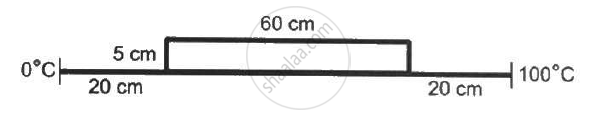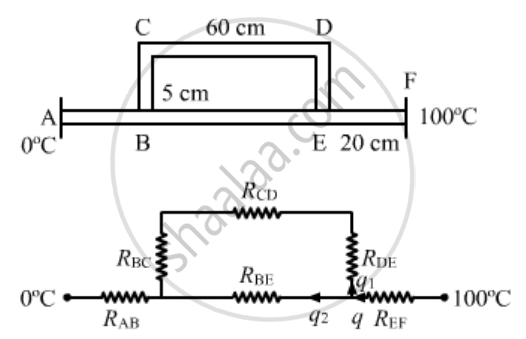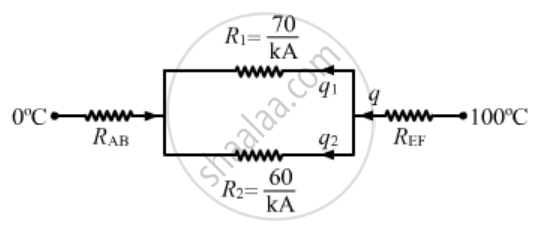Share

# Consider the Situation Shown in Figure (28-e5). the Frame is Made of the Same Material and Has a Uniform Cross-sectional Area Everywhere. Calculate the Amount of Heat Flowing per Second - Physics

ConceptHeat Transfer Conduction

#### Question

Consider the situation shown in the figure . The frame is made of the same material and has a uniform cross-sectional area everywhere. Calculate the amount of heat flowing per second through a cross section of the bent part if the total heat taken out per second from the end at 100°C is 130 J.#### SolutionR_{BC} = 1/{KA }= 1/{KA}

R_{CD} = 1/{KA }= 60/{KA}

R_{CD} = 5/{KA} ,  R_{AB} = 20/M , R_{EF} =  20/{KA}

Let ;

R_1 = R_{BC} + R_{CD} + R_{DE} = 70/{KA}

Let :

R_{BE} = 60/{KA} = R_2q = q1 + q2        ...............(1)

R1 and R2 are in parallel, so total heat across R1 and R2 will be same.

⇒ q_1R_2 = q_2R_2

q_1xx 70/{KA} = q_2xx 60/{KA}

7q_1 = 6q_2

{7q_1}/{6}= q_2

From equation (1) and (2),

q = q_1 + {7q_1}/6

q = {13q_1}/6

q=130  J
130 = 13Qq_1
q1 = 60 J/sec

Is there an error in this question or solution?

#### APPEARS IN

Solution Consider the Situation Shown in Figure (28-e5). the Frame is Made of the Same Material and Has a Uniform Cross-sectional Area Everywhere. Calculate the Amount of Heat Flowing per Second Concept: Heat Transfer - Conduction.
S